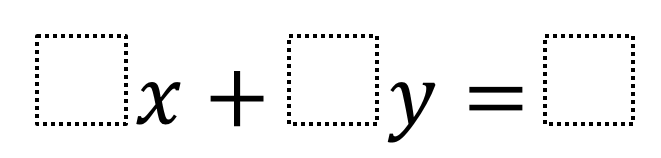Home > High School: Functions > Finding Intercepts

# Finding Intercepts

Directions:  Using the digits 1 through 9, at most one time each, fill in the boxes to create a linear equation that has an x- and y-intercept with integer values.### Hint

When finding an x – intercept, how does the coefficient of the x-variable operate with the constant value?

When finding a y-intercept, how does the coefficient of the y-variable operate with the constant value?

There are many possible solutions:

Example:

2x + 3y =6

1x + 4y = 8

3x + y =9

Source: Jeffrey Mashbitz

## Creating Sequences

Directions: Using the digits 1-9, at most one time each, complete the first three terms …

1.•